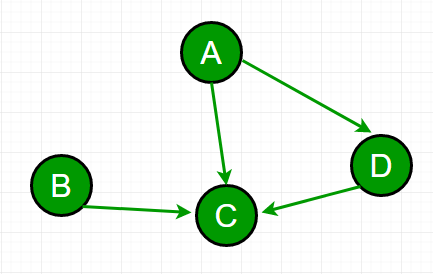Open in App
Not now

# Sum of dependencies in a graph

• Difficulty Level : Basic
• Last Updated : 08 Jul, 2022

Given a directed and connected graph with n nodes. If there is an edge from u to v then u depends on v. Our task was to find out the sum of dependencies for every node.Example:

```For the graph in diagram,
A depends on C and D i.e. 2
B depends on C i.e. 1
D depends on C i.e. 1
And C depends on none.
Hence answer -> 0 + 1 + 1 + 2 = 4```

Idea is to check adjacency list and find how many edges are there from each vertex and return the total number of edges.

Implementation:

## C++

 `// C++ program to find the sum of dependencies` `#include ` `using` `namespace` `std;`   `// To add an edge` `void` `addEdge(vector <``int``> adj[], ``int` `u,``int` `v)` `{` `    ``adj[u].push_back(v);` `}`   `// find the sum of all dependencies` `int` `findSum(vector<``int``> adj[], ``int` `V)` `{` `    ``int` `sum = 0;`   `    ``// just find the size at each vector's index` `    ``for` `(``int` `u = 0; u < V; u++)` `        ``sum += adj[u].size();`   `    ``return` `sum;` `}`   `// Driver code` `int` `main()` `{` `    ``int` `V = 4;` `    ``vector<``int` `>adj[V];` `    ``addEdge(adj, 0, 2);` `    ``addEdge(adj, 0, 3);` `    ``addEdge(adj, 1, 3);` `    ``addEdge(adj, 2, 3);`   `    ``cout << ``"Sum of dependencies is "` `         ``<< findSum(adj, V);` `    ``return` `0;` `}`

## Java

 `// Java program to find the sum of dependencies`   `import` `java.util.Vector;`   `class` `Test` `{` `    ``// To add an edge` `    ``static` `void` `addEdge(Vector adj[], ``int` `u,``int` `v)` `    ``{` `        ``adj[u].addElement((v));` `    ``}` `    `  `    ``// find the sum of all dependencies` `    ``static` `int` `findSum(Vector adj[], ``int` `V)` `    ``{` `        ``int` `sum = ``0``;` `     `  `        ``// just find the size at each vector's index` `        ``for` `(``int` `u = ``0``; u < V; u++)` `            ``sum += adj[u].size();` `     `  `        ``return` `sum;` `    ``}` `    `  `    ``// Driver method` `    ``public` `static` `void` `main(String[] args) ` `    ``{` `        ``int` `V = ``4``;` `          ``@SuppressWarnings``(``"unchecked"``)` `        ``Vector adj[] = ``new` `Vector[V];` `        `  `        ``for` `(``int` `i = ``0``; i < adj.length; i++) {` `            ``adj[i] = ``new` `Vector<>();` `        ``}` `        `  `        ``addEdge(adj, ``0``, ``2``);` `        ``addEdge(adj, ``0``, ``3``);` `        ``addEdge(adj, ``1``, ``3``);` `        ``addEdge(adj, ``2``, ``3``);` `     `  `        ``System.out.println(``"Sum of dependencies is "` `+` `                            ``findSum(adj, V));` `    ``}` `}` `// This code is contributed by Gaurav Miglani`

## Python3

 `# Python3 program to find the sum ` `# of dependencies`   `# To add an edge` `def` `addEdge(adj, u, v):`   `    ``adj[u].append(v)`   `# Find the sum of all dependencies` `def` `findSum(adj, V):` `    `  `    ``sum` `=` `0` `    `  `    ``# Just find the size at each ` `    ``# vector's index` `    ``for` `u ``in` `range``(V):` `        ``sum` `+``=` `len``(adj[u])` `        `  `    ``return` `sum`   `# Driver code` `if` `__name__``=``=``'__main__'``:`   `    ``V ``=` `4` `    ``adj ``=` `[[] ``for` `i ``in` `range``(V)]` `    `  `    ``addEdge(adj, ``0``, ``2``)` `    ``addEdge(adj, ``0``, ``3``)` `    ``addEdge(adj, ``1``, ``3``)` `    ``addEdge(adj, ``2``, ``3``)` `    `  `    ``print``(``"Sum of dependencies is"``,` `          ``findSum(adj, V))` `    `  `# This code is contributed by rutvik_56`

## C#

 `// C# program to find the sum of dependencies` `using` `System;` `using` `System.Collections;`   `class` `GFG{` `    `  `// To add an edge` `static` `void` `addEdge(ArrayList []adj, ``int` `u,` `                                     ``int` `v)` `{` `    ``adj[u].Add(v);` `}`   `// Find the sum of all dependencies` `static` `int` `findSum(ArrayList []adj, ``int` `V)` `{` `    ``int` `sum = 0;` `    `  `    ``// Just find the size at each ` `    ``// vector's index` `    ``for``(``int` `u = 0; u < V; u++)` `        ``sum += adj[u].Count;` ` `  `    ``return` `sum;` `}`   `// Driver code` `public` `static` `void` `Main(``string``[] args) ` `{` `    ``int` `V = 4;` `      `  `    ``ArrayList []adj = ``new` `ArrayList[V];` `    `  `    ``for``(``int` `i = 0; i < V; i++)` `    ``{` `        ``adj[i] = ``new` `ArrayList();` `    ``}` `    `  `    ``addEdge(adj, 0, 2);` `    ``addEdge(adj, 0, 3);` `    ``addEdge(adj, 1, 3);` `    ``addEdge(adj, 2, 3);` ` `  `    ``Console.Write(``"Sum of dependencies is "` `+` `                  ``findSum(adj, V));` `}` `}`   `// This code is contributed by pratham76`

Output

`Sum of dependencies is 4`

Time complexity: O(V) where V is number of vertices in graph.

This article is contributed by Sahil Chhabra (akku). If you like GeeksforGeeks and would like to contribute, you can also write an article using write.geeksforgeeks.org or mail your article to review-team@geeksforgeeks.org. See your article appearing on the GeeksforGeeks main page and help other Geeks.

My Personal Notes arrow_drop_up
Related Articles